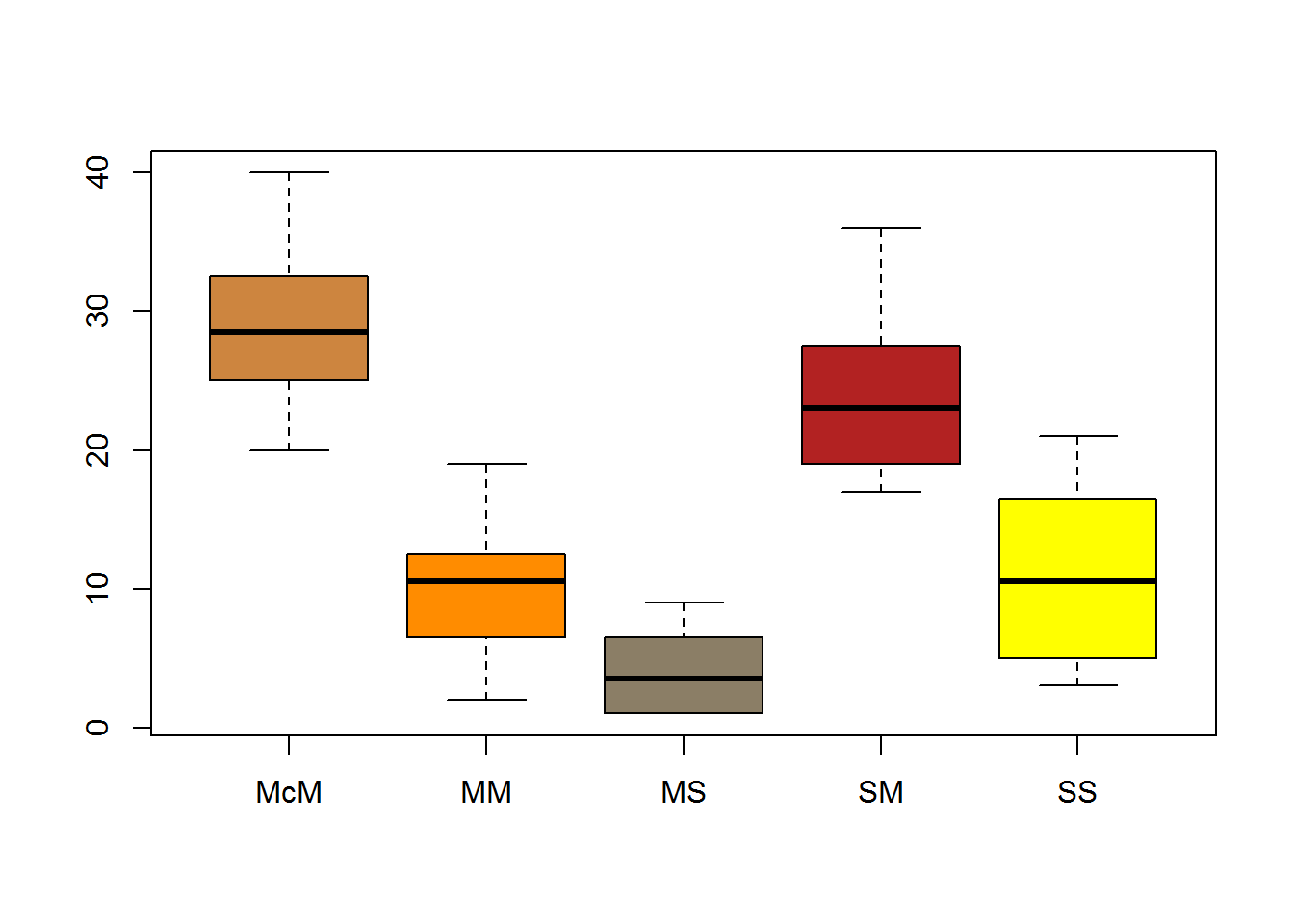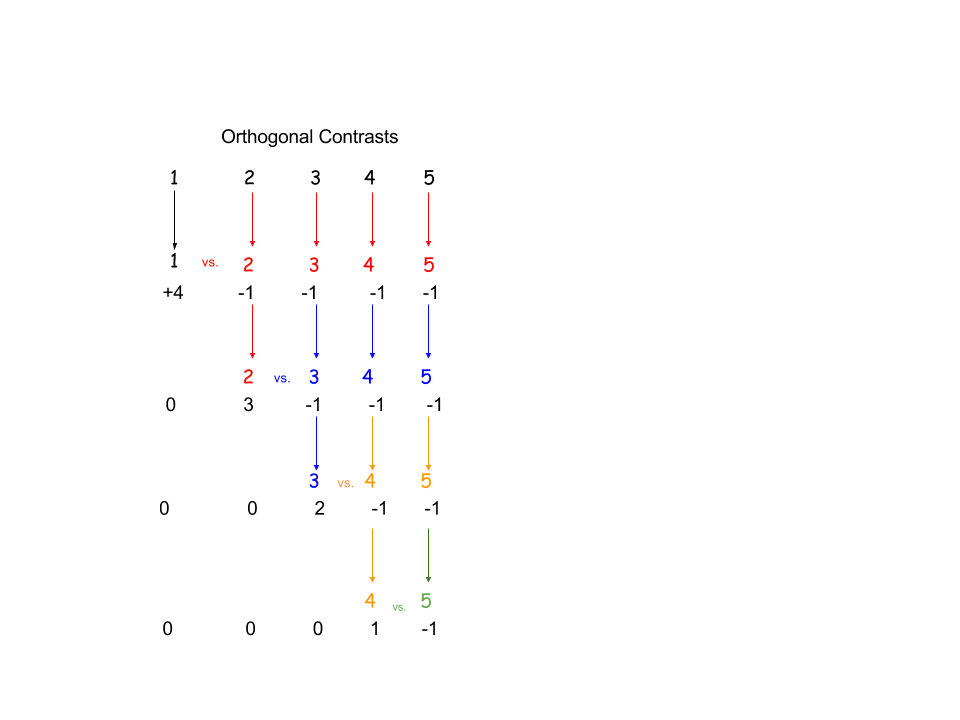### ANOVA:

Five groups of rats: MS received morphine on $$3$$ occasions followed by saline; MM received morphine on $$4$$ occasions; SS received only saline; and McM morphine in $$4$$ separate occasions, but last dose was administer in a different environment. The latency betwen liking their paws was measured after the last dose.

##   MS MM SS SM McM
## 1  3  2 14 29  24
## 2  5 12  6 20  26
## 3  1 13 12 36  40
## 4  8  6  4 21  32
## 5  1 10 19 25  20
## 6  1  7  3 18  33
## 7  4 11  9 26  27
## 8  9 19 21 17  30

We can run an ANOVA after preparing the data in long format:

require("reshape")
dat = melt(d)
colnames(dat)=c("Rat","Sequence","Latency")
summary(aov(Latency ~ Sequence, dat))
##             Df Sum Sq Mean Sq F value   Pr(>F)
## Sequence     4   3498   874.4   27.32 2.44e-10 ***
## Residuals   35   1120    32.0
## ---
## Signif. codes:  0 '***' 0.001 '**' 0.01 '*' 0.05 '.' 0.1 ' ' 1The calculations involved can be carried out manually as follows:

(SSBetween = sum(nrow(d) * (colMeans(d) - mean(d))^2))
##  3497.6
(SSWithin = sum(scale(d, center = T, scale = F)^2)) 
##  1120
(SSTotal = sum((d - mean(d))^2))  
##  4617.6
(df_btw = ncol(d) - 1)
##  4
(MeanSqBetween = SSBetween / df_btw)
##  874.4
(df_within = length(d) - ncol(d))
##  35
(MeanSqWithin = SSWithin / df_within)
##  32
(F_value = MeanSqBetween / MeanSqWithin) 
##  27.325
1 - pf(F_value, ncol(d)-1, length(d)-ncol(d))
##  2.443479e-10
summary(aov(Latency ~ Sequence, dat)) # For comparison.
##             Df Sum Sq Mean Sq F value   Pr(>F)
## Sequence     4   3498   874.4   27.32 2.44e-10 ***
## Residuals   35   1120    32.0
## ---
## Signif. codes:  0 '***' 0.001 '**' 0.01 '*' 0.05 '.' 0.1 ' ' 1

Here is the equivalent calculation with lm():

summary(lm(Latency ~ Sequence, dat))

### CONTRASTS:

A linear combination of the group means is a contrast if:

$\sum_{j = 1}^{k}a_j \bar X_j= a_1\bar X_1+ a_2 \bar X_2 +\cdots + a_k\bar X_k$

and $$\sum_{j = 1}^{k}a_j=0$$

Linear contrasts are typically a priori tests. A priori tests are planned even before the data is collected. A priori tests imply a relatively small set of comparisons. If we make all possible pairwise comparisons among several means, it doesn’t make any difference whether they was planned in advance or not. The idea is to minimize the familywize error rate. Looking at the data before deciding which comparisons will be carried out greatly predisposes to type I errors, because all the other comparisons are implicitely made to pick up the largest differences. That is why for some post hoc tests, the error rate is adjusted as though all possible comparisons had been made.

Contrasts can be set up to compare one mean to another. For example in the case of three means:

$L=1 \times \bar X_1 -1 \times \bar X_2 + 0 \times \bar X_3=\bar X_1 - \bar X_2$

They can also compare the average of $$2$$ means with a third mean:

$L=1/2\times \bar X_1 +1/2 \times \bar X_2 -1 \times \bar X_3=\frac{\bar X_1 + \bar X_2}{2}-\bar X_3$

To see the relation between the sum of squares of contrasts with the initial sum of squares “between” treatments, we can see that the sum of squares of orthogonal contrasts adds up to the SS “between”, defining the sum of squares between contrasts as:

$\Large SS_{\text{contrast}}=\frac{n\,L^2}{\sum a_j^2}$

$$n$$ corresponds to the number of cases.

For contrasts to be orthogonal their dot products have to be zero, and the number of comparisons identical to the $$df$$ for treatments. There are many sets of orthogonal contrasts, and which ones to run is up to the experimenter.For the dataset above, with $$5$$ columns, the orthogonal contrasts (dot product of zero) are:

\begin{align} [4\,\,;\,-1\,\,;\,-1\,\,;\,-1\,\,;\,-1]\\ [0\,\,;\,3\,\,;\,-1\,\,;\,-1\,\,;\,-1]\\ [0\,\,;\,0\,\,;\,2\,\,;\,-1\,\,;\,-1]\\ [0\,\,;\,0\,\,;\,0\,\,;\,1\,\,;\,-1]\\ \end{align}
M = colMeans(d)

L1 = 4 * M - 1 * M - 1 * M - 1 * M - 1 * M
SSc1 = nrow(d) * L1^2 / sum(16, 4 * (-1)^2)

L2 = 3 * M -1 * M -1 * M -1 * M
ssc2 = nrow(d) * L2^2 / (sum(9, 3 * (-1)^2))

L3 = 2 * M -1 * M -1 * M
ssc3 = nrow(d) * L3^2/ sum(4 + 2 * (-1)^2)

L4 = M - M
ssc4 = nrow(d) * L4^2 / 2

sum(SSc1, ssc2, ssc3, ssc4)
##  3497.6
(SSBetween = sum(nrow(d) * (colMeans(d) - mean(d))^2))
##  3497.6

If we take the second contrast, $$[a_1=0\,\,;a_2=3\,\,;a_3=-1\,\,;a_4=-1\,\,;a_5=-1]$$, and we change it to $$[a_1=0\,\,;a_2=1\,\,;a_3=-1/3\,\,;a_4=-1/3\,\,;a_5=-1/3]$$, the sum of squares of the contrast will be:

(L_2 = as.numeric(1 * M - 1/3 * M - 1/3 * M - 1/3 * M))
##  -11.33333
(ssc_2 = nrow(d) * L_2^2 / ((1)^2 + 3 * (-1/3)^2))
##  770.6667
# compared to:
(L2 = as.numeric(3 * M -1 * M -1 * M -1 * M))
##  -34
(ssc2 = nrow(d) * L2^2 / (sum(9, 3 * (-1)^2)))
##  770.6667

identical!

Contrast have $$1$$ degree of freedom, so that the $$F$$ value of again the second contrast, calculated as:

$\large F=\frac{\text{MS}_{\text{contrast}}}{\text{MS}_{\text{error}}} =\frac{\frac{n\,L^2}{\sum a_j^2}}{\text{MS}_{\text{error}}}$

will be:

(F = nrow(d) * L2^2 / 4) / MeanSqWithin
##  72.25

compared to t-test of M + M versus M + M

A contrast such as: $$[a_1=1\,\,;a_2=-1\,\,;a_3=0\,\,;a_4=0\,\,;a_5=0]$$, would have an $$F$$ value:

(F = (nrow(d) * (sum(M - M))^2/2) / MeanSqWithin)
##  4.5

with $$1$$ df, which corresponds to a significance level of $$F_{0.05}(1,35)=4.12$$, or qf(0.95,df1=1, df2=35). Therefore it is statistically significant.